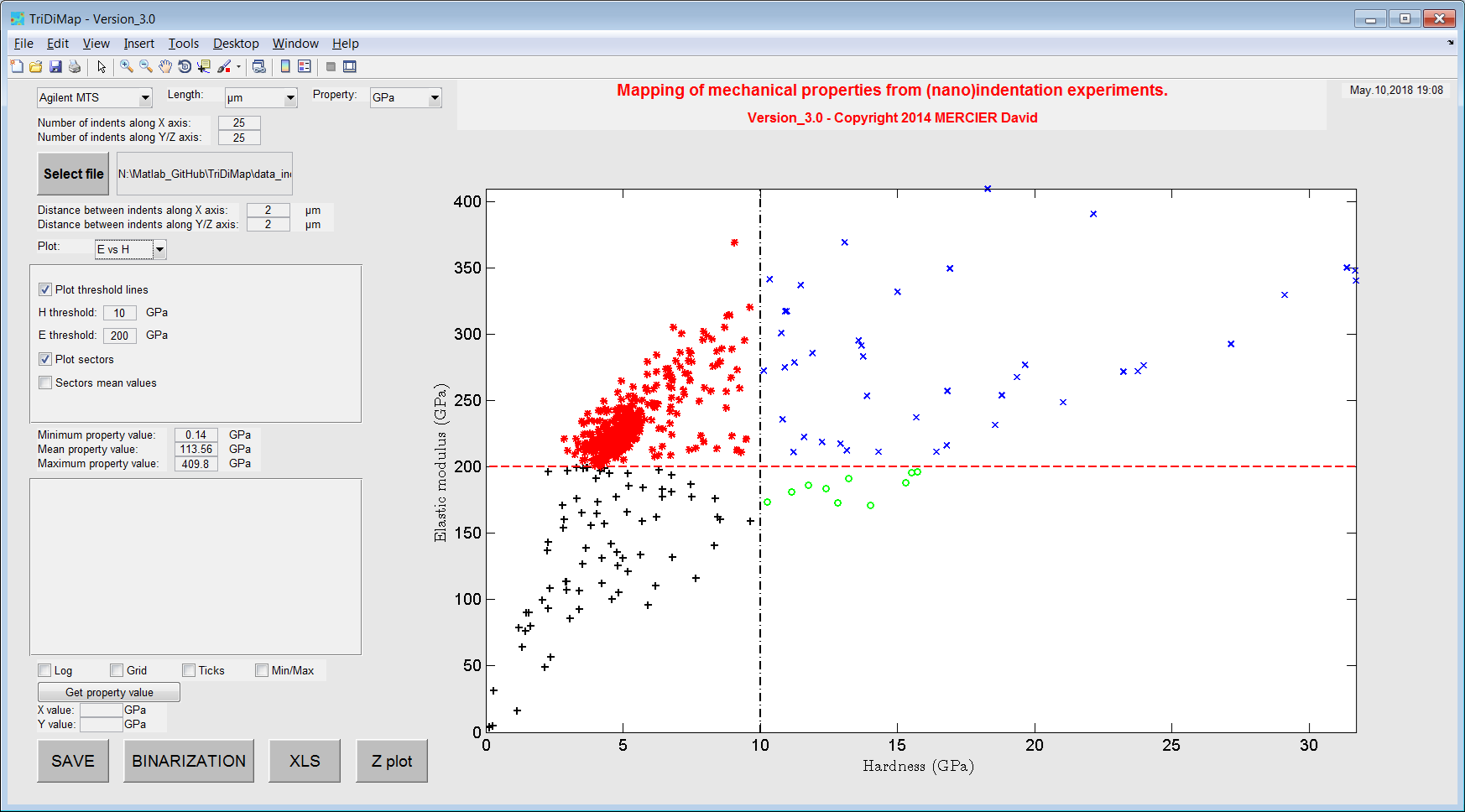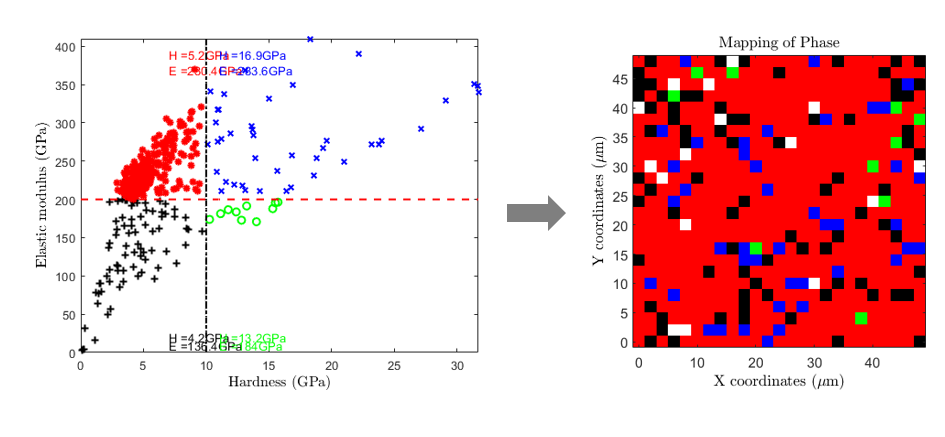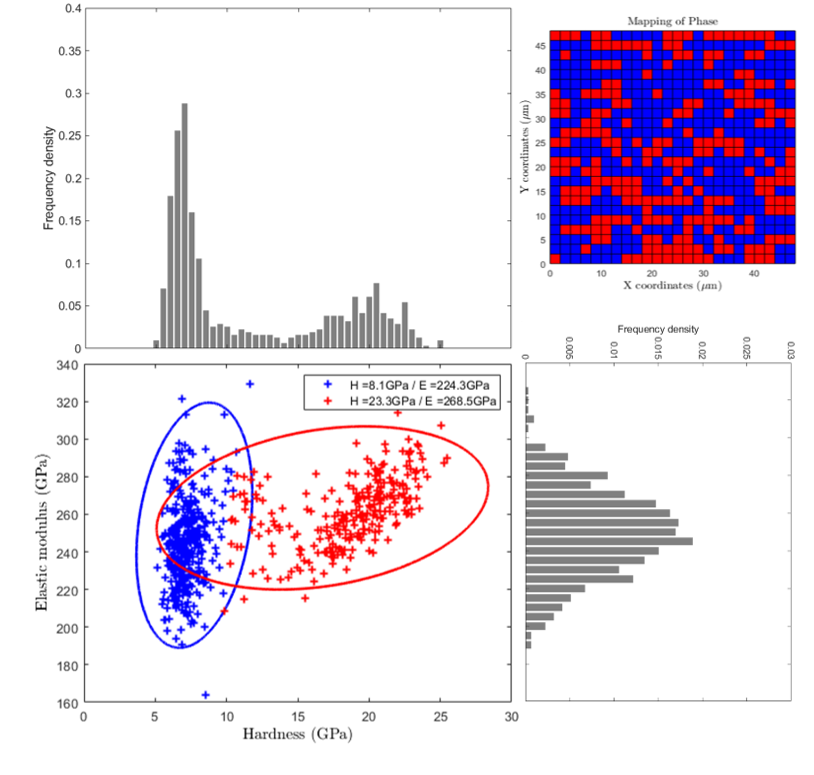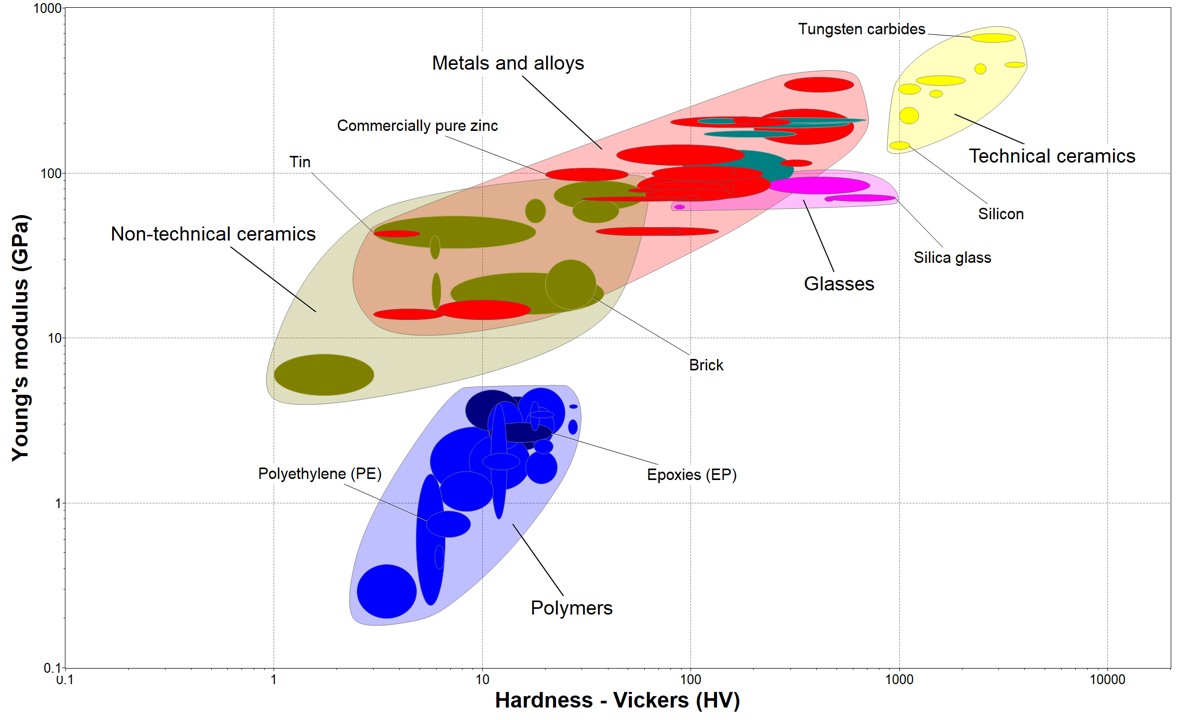Elastic modulus vs Hardness plot¶

Another way to visualize the distribution of mechanical property results is to plot for example the elastic modulus (E) values vs the hardness (H) values. Such a plot leads sometimes to the observation of families of points and the definition of “sectors” or “bubbles”, each one corresponding to a single phase (e.g. soft matrix vs hard and stiff particles).

The correlation between elastic and plastic properties has been extensively studied in the literature , ,  and .

Note

Elastic modulus is an intrinsic material property and hardness is an engineering property, which can be related to yield strength for some materials.

E-H map sectorization¶

As a first analysis of such a plot, sectors can be defined by giving an average value of elastic modulus and an average of hardness value, separating respectively by an horizontal line and a vertical line the different bubbles of points. Each sector is defined by a unique color.

Finally, average values of mechanical properties are given for each sectors directly into the graph, and a 4 color-coded map corresponding to this plot can be generated (see 2nd figure).Figure 30 Example of sectorized elastic modulus vs hardness plotFigure 31 Sectorized elastic modulus vs hardness plot with mean values and corresponding mechanical map

Clustering with Gaussian Mixture Models¶

The Gaussian mixture Models (GMM) are often used for data clustering . This method is well described in the Matlab documentation ,  and  but also in the literature .

This method is powerful to separate contribution of 2 or 3 phases (especially in the case of a soft metallic matrix with hard ceramic particles) in the cloud of experimental points . Average mechanical property values can also be extracted using this method and a 2 or 3 color map can be obtained too.

The Matlab third party code used to define clusters GMM is: GMMClustering.mFigure 32 Elastic modulus vs hardness plot with clusters of points obtained with GMM

Ashby map¶

Such plot could be seen as a conventional Ashby map , with materials families. An example of a typical Ashby map is given afterwards, using the CES Selector 2018 software . At some point, it is possible to add material reference (bulk, homogeneous, monophasic, …) values on the E-H map, in order to compare experimental data with data from the literature.Figure 33 Typical Ashby map of elastic modulus vs Vickers hardness, obtained using CES Selector software